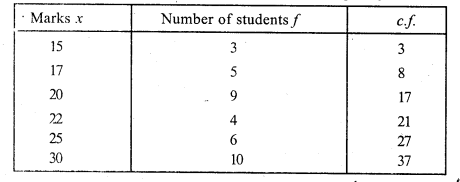# RS Aggarwal Class 7 Solutions Chapter 21 Collection and Organisation of Data Ex 21B

In this chapter, we provide RS Aggarwal Solutions for Class 7 Chapter 21 Collection and Organisation of Data Ex 21B for English medium students, Which will very helpful for every student in their exams. Students can download the latest RS Aggarwal Solutions for Class 7 Chapter 21 Collection and Organisation of Data Ex 21B Maths pdf, free RS Aggarwal Solutions Class 7 Chapter 21 Collection and Organisation of Data Ex 21B Maths book pdf download. Now you will get step by step solution to each question.

### RS Aggarwal Solutions for Class 7 Chapter 21 Collection and Organisation of Data Ex 21B Download PDF

Question 1.
Solution:
(i) Arranging in ascending order.
2, 2, 3, 5, 7, 9, 9, 10, 11
Here number of terms = 9 which is odd
Median= n+12 = 9+12 th term
= 5th term = 7
Hence median = 7
(ii) Arranging in ascending order,
6, 8, 9, 15, 16, 18, 21, 22, 25
Here, number of terms (n) = 9 which is odd
Median= n+12 = 9+12 th term
= 5th term = 16
(iii) Arranging in ascending order,
6, 8, 9, 13, 15, 16, 18, 20, 21, 22, 25 Here number of terms (n) = 11 which is odd
Median= n+12 = 11+12 th term
= 6th term = 16

Question 2.
Solution:
(i) Arranging in ascending order,
9, 10, 17, 19, 21, 22, 32, 35
Here, number of terms = 8 which is evenQuestion 3.
Solution:
First 15 odd numbers are 1, 3, 5, 7, 9, 11, 13, 15, 17, 19, 21, 23, 25, 27, 29.
Here, number of terms (n) = 15 which is odd
Median = n+12 = 15+12 th term
= 8th term = 15

Question 4.
Solution:
First 10 even numbers are 2, 4, 6, 8, 10, 12, 14, 16, 18, 20
Here, number of terms = 10 which is evenQuestion 5.
Solution:
First 50 whole numbers
0, 1, 2, 3, 4, …, 49
Here, number of terms = 50, which is evenQuestion 6.
Solution:
Arranging in ascending order,
17, 17, 19, 19, 20, 21, 22, 23, 24, 25, 26, 29, 31, 35, 40 .
Here, number of terms = 15 which is odd
Median = n+12 th term = 15+12 = 162 th
= 8th term = 23

Question 7.
Solution:
Arranging is ascending order,
31, 34, 36, 37, 40, 43, 46, 50, 52, 53
Here, number of terms = 10, which is evenQuestion 8.
Solution:
Preparing the cumulative frequency tableHere, number of terms (N) = 41, which is odd
Median = n+12 th term
= 41+12 = 422 th = 21 th term = 50kg (value of 20 to 28 = 50)
Hence median = 50kg

Question 9.
Solution:
Arranging in order and preparing the cumulative frequency table.Here, number of terms (N) = 37 which is odd.
Median = n+12 th term
= 37+12 = 382 th term
= 19th term = 22 (Value of 18 to 21 = 22)
Hence median = 22

Question 10.
Solution:
Arranging in order and then preparing its cumulative frequency table :Here, number of terms (N) = 50, which is evenHence median = 154.5 cm

All Chapter RS Aggarwal Solutions For Class 7 Maths

—————————————————————————–

All Subject NCERT Exemplar Problems Solutions For Class 7

All Subject NCERT Solutions For Class 7

*************************************************

I think you got complete solutions for this chapter. If You have any queries regarding this chapter, please comment on the below section our subject teacher will answer you. We tried our best to give complete solutions so you got good marks in your exam.

If these solutions have helped you, you can also share rsaggarwalsolutions.in to your friends.